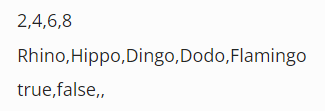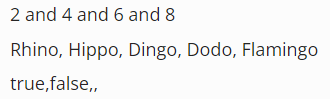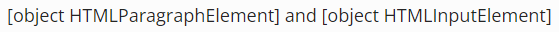Lesson Wednesday

In this lesson, we'll review how to print the contents of an array to a webpage. To do this, we'll use the webpage you are on right now!

## Printing an Array of Primitives

To print an array of primitives to a webpage, we can set the value of the `innerText` property of a paragraph element to an array. Let's try this in the DevTools console. In this example, we'll create code that outputs to the section below titled "output".

First, we'll create three paragraph elements and we'll add each to the hidden div lower on this page. We'll use 3 paragraph elements, so we can see all examples side by side.

``````> const div = document.querySelector("div#lesson-ex");
undefined
> div;
<div id="lesson-ex"></div>
> const p1 = document.createElement("p");
undefined
> const p2 = document.createElement("p");
undefined
> const p3 = document.createElement("p");
undefined
> div.append(p1, p2, p3);
``````

Great! Now we have three empty P tag that we can use to print the contents of three arrays. Next let's create a few arrays and try outputting them below.

``````> const numAr = [2,4,6,8];
> const stringAr = ["Rhino", "Hippo", "Dingo", "Dodo", "Flamingo"];
> const morePrimitives = [true, false, undefined, null];
``````

Now let's try printing these to the output below.

``````> p1.innerText = numAr;
> p2.innerText = stringAr;
> p3.innerText = morePrimitives;
``````

As we'll see below in the output section as well as in the image that follows, the contents of the array is printed and each element is separated with a comma. This is standard when using this method of printing the contents of an array of primitives: each element is always separated by a comma. If we want to add a space in between the commas of each value, then we'll have to do an extra step.

Notice, too, that `undefined` and `null` are represented as nothing on the page, but they are still separated by a comma.### Output

There's a div below this line where we'll print the examples arrays from this lesson.

### Formatting Array Values to Print in a Paragraph

To format our array values, we can use the built-in array method `Array.prototype.join()`. This method will turn an array into a string, separating each element by the argument that we pass into the method. Let's see some examples.

``````> p1.innerText = numAr.join(" and ");
> p2.innerText = stringAr.join(", ");
> p3.innerText = morePrimitives.join();
``````

As we can see in the image below and the "output" section above, the argument that we pass into the `Array.prototype.join()` method determines how our array is rejoined together back into a string.## Printing an Array of Objects

We can't print an array of objects (like HTML element objects) to a webpage like we did with an array of primitives. Let's see an example.

``````> const pTag = document.createElement("p");
undefined
> const input = document.createElement("input");
undefined
> const objArray = [pTag,input];
undefined
> p1.innerText = objArray.join(" and ");
``````

If we look up in the "output" section above, or the image below, we'll see how our HTML element objects don't print like primitives do: `[object HTMLParagraphElement] and [object HTMLInputElement]`. Note that this is true for any object, not just HTML element objects.So what can we do about this? To get the information of an object printed to the webpage, we need to print the value of properties that are set to primitive values, like our P tag's `innerText` property or the input's `value` property.

A good example of looping through an array of HTML element objects is the Transportation Survey project that we used to learn how to implement checkboxes earlier in this course section. In that project, we created new paragraph elements for every transportation option that the user checked, and added each paragraph to the DOM in a 'results' section.